Home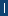Contact Us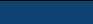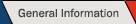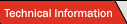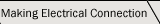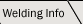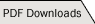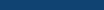Currents Voltage Circuits Power Supplies Calculator

# Series & Paralell Circuits

There are two main differences between series and parallel circuits; total voltage, and total current. Series circuits have higher voltage lower current, and parallel circuits have higher current with lower voltage.

### Parallel Circuits:

Voltage is dependent on the longest length of Powercore NOT the total number of feet of Powercore.

Total volts = (Volts/foot) x (maximum length in feet)

Current is the current per core, multiplied by the total number of Powercores to be welded.

Total Current = 6 a x ( Total No. of Powercores)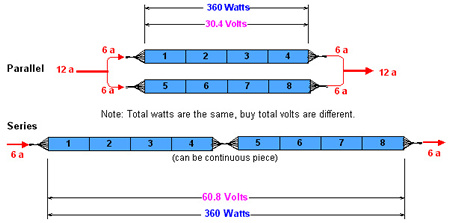### Series Circuits:

Voltage is dependent on the longest length of Powercore ( the total number of feet in this case ).

Total volts = (Volts/foot) x (total number of feet)

Current is the minimum current required (6 amps).

Welding Parallel & Series Loop Circuits:

When making a parallel loop circuit, the Powercore welding rod can actually touch without shorting out. The Powercore will not short out because there is an equal potential difference between the wires at the same point along their length.

In a series loop circuit, the ends of the Powercore must be placed 1/16" (2mm) apart to prevent shorting. During the welding process the plastic around the wires will migrate together to form an air tight joint, but the wires will remain in the same location.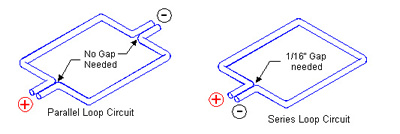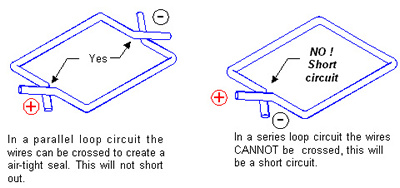For easy reference, we have provided all the above information in PDF format for you to download.

 Privacy PolicyCopyright ©2005 Powercore International, Ltd.